Hans Walser, 

Geometric Sequence and Triangles

Motivation: M. N. D., N.

Let a, b, c the sides of a triangle ABC such that(in this order) is a geometric sequence. This means that we have a ratio r such that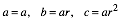.

Figure 1 gives an example with the ratio r = 1.2.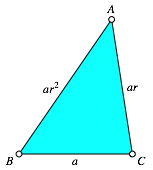Fig. 1: Ratio r = 1.2

We will see that the Golden Section plays an important role in special cases of these triangles.

# 2        Special triangles

## 2.1      Equilateral triangle

In the trivial case of r = 1 we have the equilateral triangle (Fig. 2).Fig. 2: Equilateral triangle

## 2.2      Right triangles

For r > 1 we have a < b < c. The theorem of Pythagoras yields:The Golden Section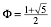comes in (Walser 2001 and Walser 2013). We get the large Right Golden Triangle of Figure 3.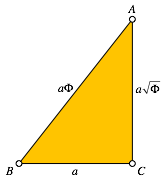Fig. 3: Large Right Golden Triangle

For r < 1 we have a > b > c. The theorem of Pythagoras yields in this case:We get the small Right Golden Triangle (Fig. 4).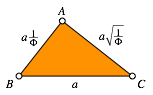Fig. 4: Small Right Golden Triangle

The small Golden Triangle fits into the large Golden Triangle (Fig. 5). It has the same shape like the large Right Golden Triangle, but a different orientation.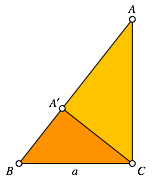Fig. 5: Both Right Golden triangles

# 3        General

## 3.1      Range of the ratio r

The triangle inequality gives the condition a + b > c. HenceOn the other side we have a < b + c. That means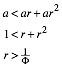Therefore we have the range.

Note that the ratios of the small Right Golden Triangle, the equilateral triangle, and the large Right Golden Triangle are within this range:Together with the range bounds they are a geometric sequence.

## 3.2      Two fix points

For the general triangle we let two of the three vertices of the triangle fix and study the locus of the third point. There are three cases:

(i)         B and C fix, A variable

(ii)       C and A fix, B variable

(iii)      A and B fix, C variable

### 3.2.1    B and C fix

In a Cartesian coordinate system we choose,, and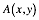(Fig. 6). For the sides of the triangle we get:The geometric sequence condition is:This yields the implicit equation for the locus of A:or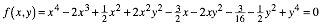We have a curve of degree 4.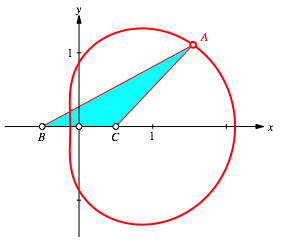Fig. 6: B and C fix

For x = 0 we find the equilateral triangle (Fig. 7).Fig. 7: Equilateral triangle

Note that the side AB of the equilateral triangle is tangential to the curve. For the proof we use the gradient:The vector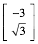is orthogonal to the curve, but also orthogonal to the side AB of the equilateral triangle.

Forwe get the large Right Golden triangle (Fig. 8).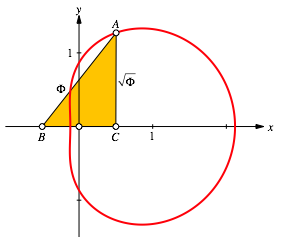Fig. 8: Right Golden Triangle

We find also the small Right Golden Triangle (Fig. 9). Here we have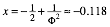Fig. 9: Right Golden Triangle

Finally we find the Golden Section in different cases (Fig. 10 and 11). The major is indicated in blue and the minor in red.Fig. 10: Golden Section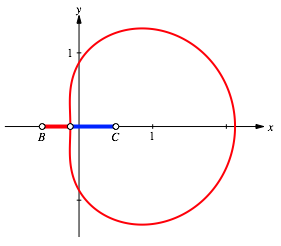Fig. 11: Golden Section

### 3.2.2    C and A fix

Now we chooseand(Fig. 12).

For the sides of the triangle we get:The geometric sequence condition is again:This yields the implicit equation for the locus of B: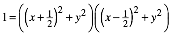Fig. 12: C and A fix

The curve looks like an ellipse, but this cannot be, since it is a curve of degree 4. Nevertheless the difference to the ellipse with the same semi axes is very small (Fig. 13).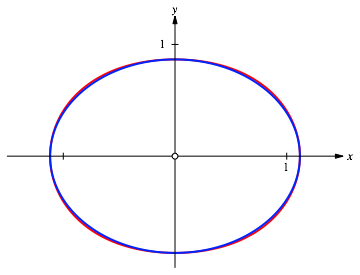Fig. 13: Curve compared with ellipse

Again we find an equilateral triangle (Fig. 14), Right Golden triangles (Fig. 15) and the golden Section (Fig. 16).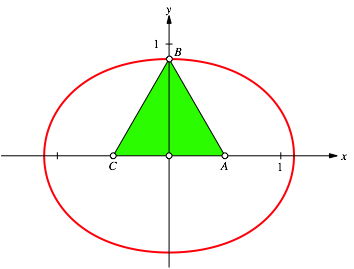Fig. 14: Equilateral triangleFig. 15: Right Golden Triangle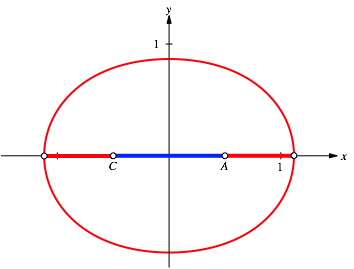Fig. 16: Golden Section

### 3.2.3    A and B fix

Now we chooseand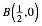(Fig. 17).

For the sides of the triangle we get:The geometric sequence condition is again: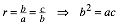This yields the implicit equation for the locus of C: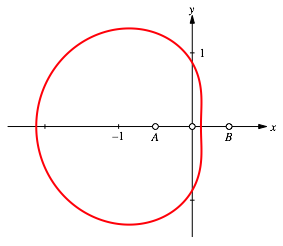Fig. 17: A and B fix

The curve is symmetric to the curve of Figure 6.

## 3.3      The three curves

Figure 18 shows the three curves in a triangular lattice. We see a lot of Golden Sections.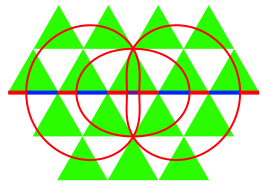Fig. 18: The tree curves

References

Walser, Hans (2001): The Golden Section. Translated by Peter Hilton and Jean Pedersen. The Mathematical Association of America 2001. ISBN 0-88385-534-8.

Walser, Hans (6. Auflage). (2013). Der Goldene Schnitt. Mit einem Beitrag von Hans Wußing über populärwissenschaftliche Mathematikliteratur aus Leipzig. Leipzig: Edition am Gutenbergplatz. ISBN 978-3-937219-85-1.